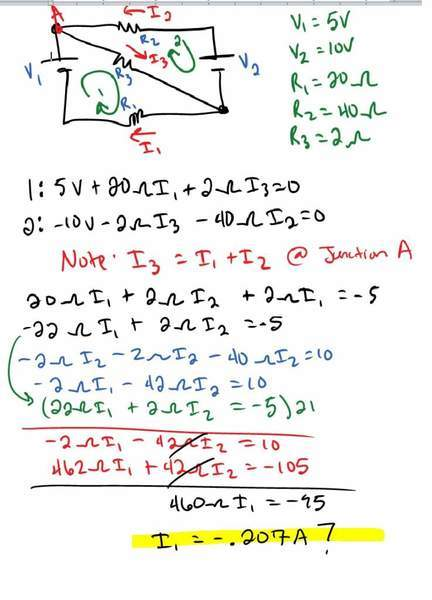# Negative current?

Sastronaut

## Homework Statement

Calculate the current through R3.

V=IR

## The Attempt at a Solution

my attempt is attached. My question revolves around my answer...is a negative current a legitimate answer? What does it mean?

#### Attachments

•phys.jpg
29.8 KB · Views: 414

## Answers and Replies

Homework Helper
Gold Member
It is a legitimate answer provided you show a direction arrow (which you have done). It means that the current is not travelling in the direction indicated by your direction arrow. It is going in the opposite direction.

Homework Helper
Gold Member
Looking at your loop equations, they seem a bit suspect. If you are summing the changes in voltage across each element along a loop, equating the sum to zero, you should get something different.

Homework Helper

## Homework Statement

Calculate the current through R3.

V=IR

## The Attempt at a Solution

my attempt is attached. My question revolves around my answer...is a negative current a legitimate answer? What does it mean?

Your equations 1 and 2 are wrong. Note, that the potential decreases in the direction of the current.

ehild

Sastronaut
What should my equation for loop 1 be?

Homework Helper
You start at the negative pole of the battery, and follow the change of potential in the direction, indicated by the green arrow. The potential increases by 5 V traversing the battery. Then it decreases by I3R3 along R3. Then again, it decreases by R1I1, when you return to your initial position. The net change of potential is zero: 5-I3R3-I1R1=0.
Do the same for the other loop. Note that going in direction opposite to the current, the potential would increase.

ehildSastronaut
okay I think I am following you so for loop 2 the equation is then -10V+I3R3+I2R2=0?

Homework Helper
okay I think I am following you so for loop 2 the equation is then -10V+I3R3+I2R2=0?

Yes.

ehild